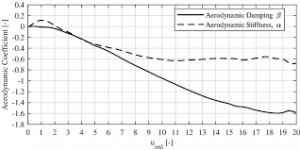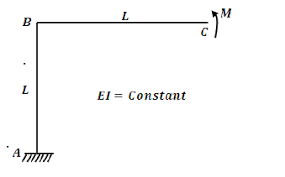## How to Calculate and Solve for Turbine Stiffness | Irrigation Water RequirementThe image above represents turbine stiffness.

To compute for turbine stiffness, three essential parameters are needed and these parameters are Modulus of Elasticity for Tubing (E), Moment of Inertia of Tubing (I) and Mean Radius of Tubing (r).

The formula for calculating turbine stiffness:

σ = EI /

Where:

σ = Turbine Stiffness
E = Modulus of Elasticity for Tubing
I = Moment of Inertia of Tubing
r = Mean Radius of Tubing

Let’s solve an example;
Find the turbine stiffness when the modulus of elasticity for tubing is 12, the moment of inertia of tubing is 10 and the mean radius of tubing is 4.

This implies that;

E = Modulus of Elasticity for Tubing = 12
I = Moment of Inertia of Tubing = 10
r = Mean Radius of Tubing = 4

σ = EI /
σ = (12)(10) / (4)³
σ = 120 / 64
σ = 1.875

Therefore, the turbine stiffness is 1.875.

Calculating for the Modulus of Elasticity for Tubing when the Turbine Stiffness, the Moment of Inertia of Tubing and the Mean Radius of Tubing is Given.

E = σ x r³ / I

Where;

E = Modulus of Elasticity for Tubing
σ = Turbine Stiffness
I = Moment of Inertia of Tubing
r = Mean Radius of Tubing

Let’s solve an example;
Find the modulus of elasticity for tubing when the turbine stiffness is 20, the moment of inertia of tubing is 10 and the mean radius of tubing is 2.

This implies that;

σ = Turbine Stiffness = 20
I = Moment of Inertia of Tubing = 10
r = Mean Radius of Tubing = 2

E = σ x r³ / I
E = 20 x 2³ / 10
E = 20 x 8 / 10
E = 160 / 10
E = 16

Therefore, the modulus of elasticity for tubing is 16.

## How to Calculate and Solve for Horizontal Deflection |Irrigation Water RequirementThe image above represents horizontal deflection.

To compute for horizontal deflection, seven essential parameters are needed and these parameters are Deflection Lag Factor (Dt), Bedding Angle Factor (K), Soil Load per Unit Length (W), Modulus of Elasticity of Tubing (E), Moment of Inertia of Tubing (I), Mean Radius of Tubing (r) and Modulus of Soil Reaction (E’).

The formula for calculating horizontal deflection:

Δx = DtKW / [EI/r³] + 0.061E’

Where:

Δx = Horizontal Deflection
Dt = Deflection Lag Factor
K = Bedding Angle Factor
W = Soil Load per Unit Length
E = Modulus of Elasticity of Tubing
I = Moment of Inertia of Tubing
r = Mean Radius of Tubing
E’ = Modulus of Soil Reaction

Let’s solve an example;
Find the horizontal deflection when the deflection lag factor is 6, the bedding angle factor is 4, the soil load per unit length is 9, the modulus of elasticity of tubing is 10, the moment of inertia of tubing is 2, the mean radius of tubing is 11 and the modulus of soil reaction is 8.

This implies that;

Dt = Deflection Lag Factor = 6
K = Bedding Angle Factor = 4
W = Soil Load per Unit Length = 9
E = Modulus of Elasticity of Tubing = 10
I = Moment of Inertia of Tubing = 2
r = Mean Radius of Tubing = 11
E’ = Modulus of Soil Reaction = 8

Δx = DtKW / [EI/r³] + 0.061E’
Δx = (6)(4)(9) / [(10)(2)/(11)³] + 0.061(8)
Δx = 216 / [20/1331] + 0.488
Δx = 216 / [0.015] + 0.488
Δx = 216 / 0.50
Δx = 429.40

Therefore, the horizontal deflection is 429.40.